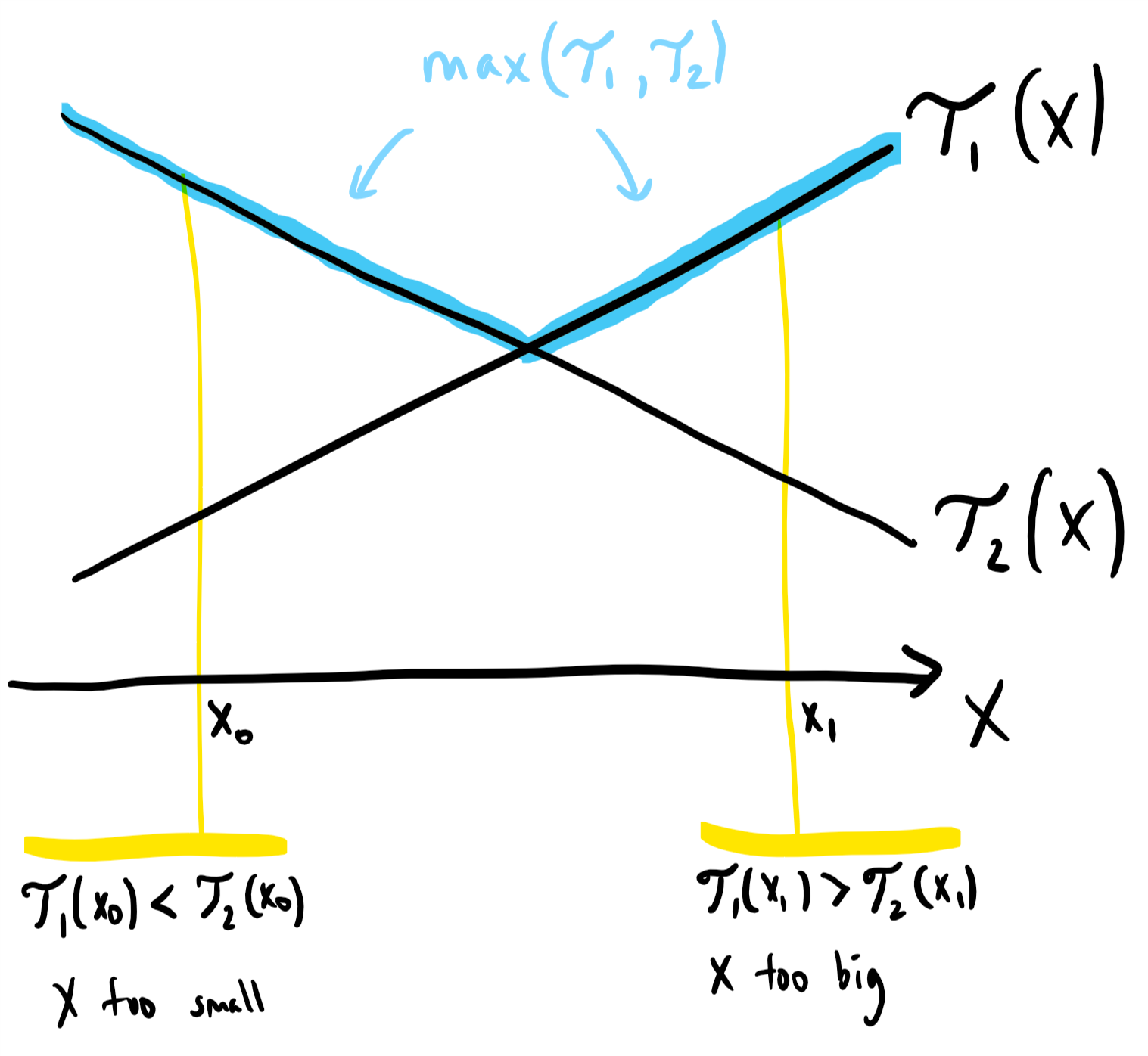## Solution

Intuition

It's natural to attempt dynamic programming, as we encounter similar subproblems. Our state is (K, N): K eggs and N floors left. When we drop an egg from floor X, it either survives and we have state (K, N-X), or it breaks and we have state (K-1, X-1).

This approach would lead to a algorithm, but this is not efficient enough for the given constraints. However, we can try to speed it up. Let dp(K, N) be the maximum number of moves needed to solve the problem in state (K, N). Then, by our reasoning above, we have:

Now for the key insight: Because is a function that is increasing on , the first term in our expression is an increasing function on , and the second term is a decreasing function on . This means that we do not need to check every to find the minimum -- instead, we can binary search for the best .

AlgorithmContinuing our discussion, if , then the value chosen is too small; and if , then is too big. However, this argument is not quite correct: when there are only two possible values of , we need to check both.

Using the above fact, we can use a binary search to find the correct value of more efficiently than checking all of them.

Complexity Analysis

• Time Complexity: .

• Space Complexity: .

#### Approach 2: Dynamic Programming with Optimality Criterion

Intuition

As in Approach 1, we try to speed up our algorithm. Again, for a state of eggs and floors, where is the answer for that state, we have:

Now, suppose is the smallest for which that minimum is attained: that is, the smallest value for which

The key insight that we will develop below, is that is an increasing function in .The first term of our expression, , is increasing with respect to , but constant with respect to . The second term, , is decreasing with respect to , but increasing with respect to .

This means that as increases, the intersection point of these two lines is increasing, as we can see in the diagram.

Algorithm

Perform "bottom up" dynamic programming based on the recurrence below, keeping track of . Again:

When we want to find , instead of searching for from , we only have to search through .

Actually, (as illustrated by the diagram,) if ever the next is worse than the current , then we've searched too far, and we know our current is best for this .

Complexity Analysis

• Time Complexity: .

• Space Complexity: .

#### Approach 3: Mathematical

Intuition

Let's ask the question in reverse: given moves (and eggs), what is the most number of floors that we can still "solve" (find with certainty)? Then, the problem is to find the least for which . Because more tries is always at least as good, is increasing on , which means we could binary search for the answer.

Now, we find a similar recurrence for as in the other approaches. If in an optimal strategy we drop the egg from floor , then either it breaks and we can solve lower floors (floors ); or it doesn't break and we can solve higher floors (floors ). In total,

Also, it is easily seen that when , and when .From here, we don't need to solve the recurrence mathematically - we could simply use it to generate all possible values of .

However, there is a mathematical solution to this recurrence. If , [the difference between the th and th term,] then subtracting the two equations:

we get:

This is a binomial recurrence with solution , so that indeed,

Alternative Mathematical Derivation

Alternatively, when we have tries and eggs, the result of our throws must be a -length sequence of successful and failed throws, with at most K failed throws. The number of sequences with failed throws is , the number of sequences with failed throw is etc., so that the number of such sequences is .

Hence, we can only distinguish at most these many floors in tries (as each sequence can only map to 1 answer per sequence.) This process includes distinguishing , so that the corresponding value of is one less than this sum.

However, this is also a lower bound for the number of floors that can be distinguished, as the result of a throw on floor will bound the answer to be either at most or greater than . Hence, in an optimal throwing strategy, each such sequence actually maps to a unique answer.

Algorithm

Recapping our algorithm, we have the increasing [wrt ] function , and we want the least so that . We binary search for the correct .

To evaluate quickly, we can transform the previous binomial coefficient to the next (in the summand) by the formula .

Complexity Analysis

• Time Complexity: .

• Space Complexity: .